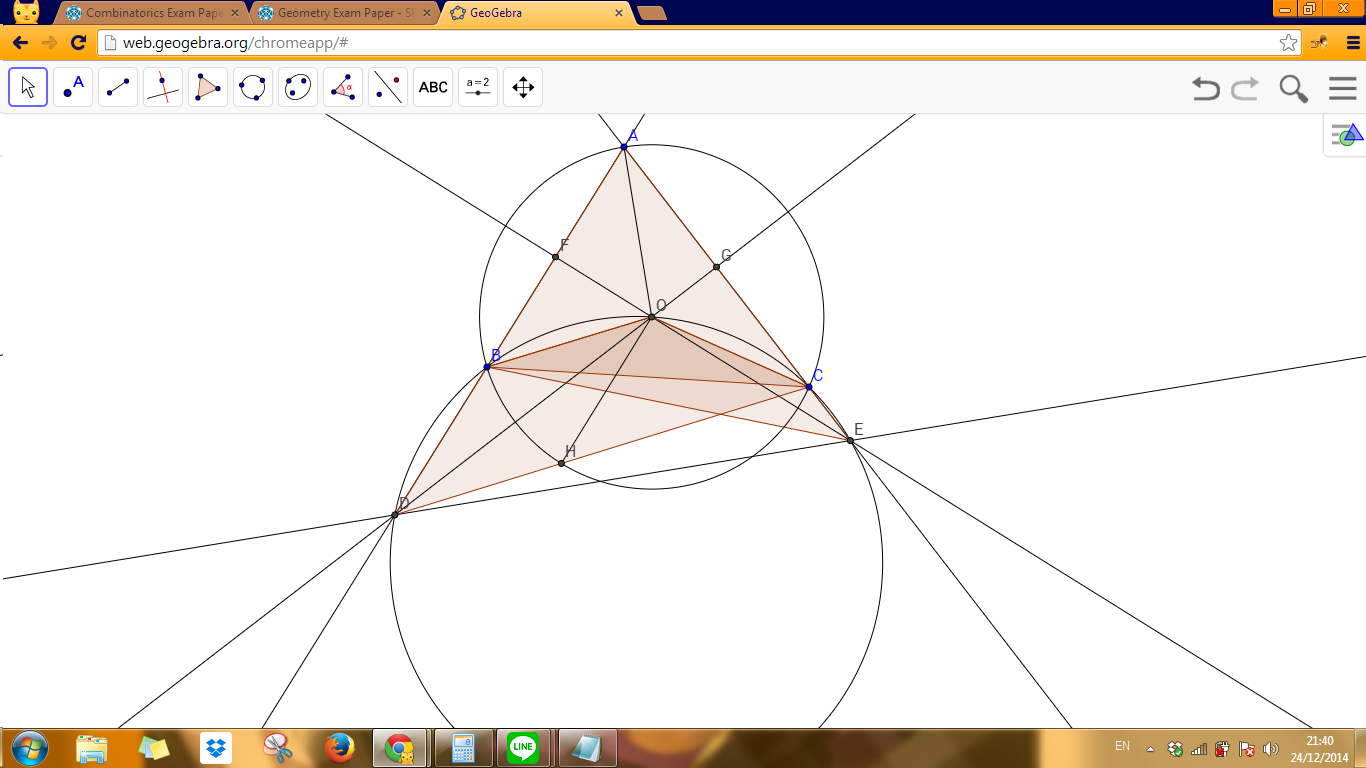# Geometry Exam Paper

Junior Exam J2

Each question is worth 7 marks.

Time: 4 hours

No books, notes or calculators allowed.

Note: You must prove your answer.

Q1

Let $AB$ be the diameter of circle $\Gamma$. Let $C$ be a point on line $AB$ outside $\Gamma$. A tangent from $C$ touches $\Gamma$ at point $N$. The bisector of $\angle ACN$ intersects segments $AN$ and $BN$ at points $P$ and $Q$, respectively.

Prove that $PN$ = $QN$.

Q2

Let $ABCD$ be a trapezium whose parallel sides are $BC$ and $AD$. Let $O$ be the intersection of the trapezium's diagonals $AC$ and $BD$. Suppose further that $CD = AO$, $BC = DO$ and that $CA$ is the bisector of $\angle BCD$.

What is the value of $\angle ABC$?

Q3

Point $P$ is situated on the hypotenuse $AB$ of right-angled triangle $ABC$, and satisfies

$PB : PC : PA = 1 : 2 : 3$

Calculate $BC : AC : AB$.

Q4

Points $D$ and $E$ lie on sides $AC$ and $BC$, respectively, of triangle $ABC$. It is known that $\angle BDE = 90^{\circ}$ and $AD = AB = BE$.

Prove that $AB + AC = 2BC$.

Q5

Let $O$ be the circumcentre of acute triangle $ABC$. A circle passing through points $B$, $O$ and $C$ intersects line $AB$ for a second time at point $D$ and intersects line $AC$ for a second time at point $E$.

Prove that lines $AO$ and $DE$ are perpendicular.Note by Sharky Kesa
6 years, 5 months ago

This discussion board is a place to discuss our Daily Challenges and the math and science related to those challenges. Explanations are more than just a solution — they should explain the steps and thinking strategies that you used to obtain the solution. Comments should further the discussion of math and science.

When posting on Brilliant:

• Use the emojis to react to an explanation, whether you're congratulating a job well done , or just really confused .
• Ask specific questions about the challenge or the steps in somebody's explanation. Well-posed questions can add a lot to the discussion, but posting "I don't understand!" doesn't help anyone.
• Try to contribute something new to the discussion, whether it is an extension, generalization or other idea related to the challenge.
• Stay on topic — we're all here to learn more about math and science, not to hear about your favorite get-rich-quick scheme or current world events.

MarkdownAppears as
*italics* or _italics_ italics
**bold** or __bold__ bold
- bulleted- list
• bulleted
• list
1. numbered2. list
1. numbered
2. list
Note: you must add a full line of space before and after lists for them to show up correctly
paragraph 1paragraph 2

paragraph 1

paragraph 2

[example link](https://brilliant.org)example link
> This is a quote
This is a quote
    # I indented these lines
# 4 spaces, and now they show
# up as a code block.

print "hello world"
# I indented these lines
# 4 spaces, and now they show
# up as a code block.

print "hello world"
MathAppears as
Remember to wrap math in $$ ... $$ or $ ... $ to ensure proper formatting.
2 \times 3 $2 \times 3$
2^{34} $2^{34}$
a_{i-1} $a_{i-1}$
\frac{2}{3} $\frac{2}{3}$
\sqrt{2} $\sqrt{2}$
\sum_{i=1}^3 $\sum_{i=1}^3$
\sin \theta $\sin \theta$
\boxed{123} $\boxed{123}$

## Comments

Sort by:

Top Newest

Q5 (Not completed, need a sleep)Construct $\overline{DO},\overline{EO}$ intersect $\overline{AC},\overline{AB}$ at point $G,F$ respectively. (sorry for someone with OCD. (not in the problem!))

We know that $\square BDCO, \square CEBO$ are concyclic, we get

$B\hat{D}O = B\hat{C}O = B\hat{E}O = O\hat{D}C$ and

$C\hat{E}O = C\hat{B}O = B\hat{C}O = B\hat{E}O$. (extra: everything above is equal!)

** Need to prove $\overline{OF} \perp \overline{AB}$ and $\overline{OG} \perp \overline{AC}$.

Consider $\triangle AEF$; by definition of orthocenter, we get $\overline{OA} \perp \overline{EF}$.

- 6 years, 5 months ago

Log in to reply

Q1: By Alternate Segment theorem $\pi-\angle ANC=\angle NBA=\pi-\angle CBN$ so we deduce $\triangle CBQ\sim\triangle PNC$. Hence $\angle CPN=\angle BQC=\angle NQP$ implying $PN=QN$.

Q5: Let the perpendicular bisector of $AC$ intersect $AB$ at $D'$. We have $\measuredangle BD'C=\measuredangle AD'C=2\measuredangle BAC=\measuredangle BOC$ meaning $BCOD'$ cyclic so $D'=D$. Similarly $E'=E$. Now in $\triangle ADE$ we have $O$ orthocenter, the result thus follows. (Angles are directed mod $\pi$)

- 6 years, 5 months ago

Log in to reply

a circle has two types of tangents.which one is the largest?

- 5 years, 10 months ago

Log in to reply

Finally cracked question 4. My solution is as follows : What we have to prove is equivalent to proving that DC=2EC. Thus we proceed as follows. Drop a perpendicular from A to BD and let it meet BD at G and BC at F. Notice that ABD is an isosceles triangle and hence BG=GD. Observe that GF is parallel to DE. Hence BF=FE. Now we have triangle CED similar to triangle CFA. Thus, CD/DA=CE/EF. Use the fact that AD=2EF. Hence obtain that DC=2.EC Hence proved

- 5 years, 5 months ago

Log in to reply

I am also done with question 3. Denote by O the midpoint of AB. Notice that OP=OR=R/2 , PC=R and hence apply Appolonius Theorem on triangle OBC with the median as PC. We get a(i.e. BC)=R(1.5)^0.5 We also know that c=2R. Hence by Pythagoras theorem we can find b and hence we can get the ratio of the sides.

- 5 years, 5 months ago

Log in to reply

×

Problem Loading...

Note Loading...

Set Loading...